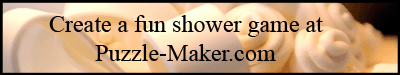Military & Political Leaders, Continued ``` 6. O A H B O A N Z E M K L A S J Q 3 0 , O A S M K A E C O W M G J Q O W , Z O K A E C Z J O Q C ; O A S O A H B O A N Z E M K E X J Q 3 0 , O A S M K A E C O F E A K J Q X O C M X J , Z O K A E G Q O M A K . - K M Q N M A K C E A F Z L Q F Z M W W ``` ```  7. K T I S A X J V T N I J Q J D A J C , Y N H Q J X J I K T I S J H H M J A I Q E D J C . - W T M Q K . G J Q Q J B V ``` ```  8. Q N Q A L W L Z A H - N X G L F , A H R C F Q M L A L X W Q B S Z Y Q P H B L ? - X M W X Y X E C Q B G H C B ``` ```  9. O R J K K M B M K C K J J K C Z J S M P P M F L W C H M A J X J Q H E R R E Q C L A M C H ; O A E R C M B M K C K J J K C Z J E R R E Q C L A M C H M A J X J Q H S M P P M F L W C H . - K M Q N M A K C E A F Z L Q F Z M W W ```